# Uniswap v3 详解（二）：创建交易对/提供流动性

Posted on March 29, 2021

## 创建交易对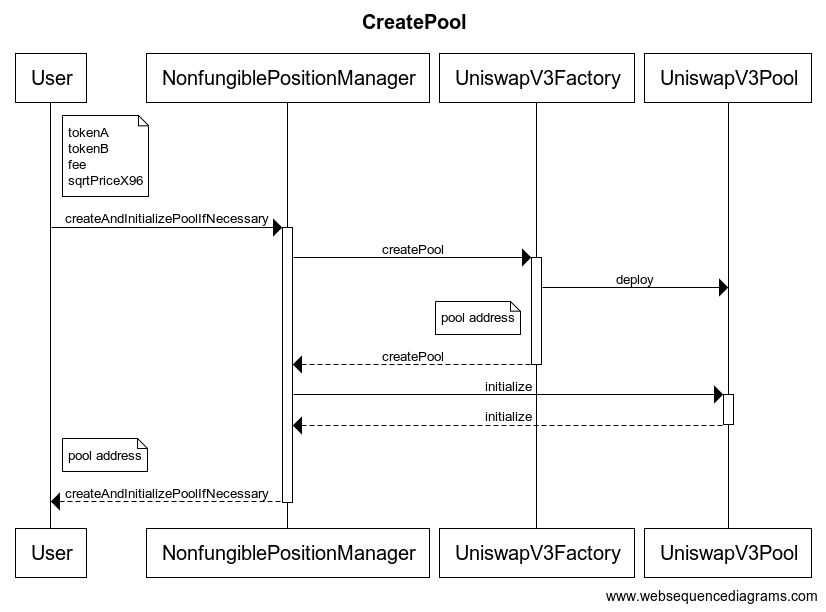NonfungiblePositionManager 合约内部通过调用 UniswapV3FactorycreatePool 方法完成交易对的创建，然后对交易对进行初始化，初始化的作用就是给交易对设置一个初始的价格。

createAndInitializePoolIfNecessary 如下：

function createAndInitializePoolIfNecessary(
uint24 fee,
uint160 sqrtPriceX96
) external payable returns (address pool) {
pool = IUniswapV3Factory(factory).getPool(tokenA, tokenB, fee);

pool = IUniswapV3Factory(factory).createPool(tokenA, tokenB, fee);
IUniswapV3Pool(pool).initialize(sqrtPriceX96);
} else {
(uint160 sqrtPriceX96Existing, , , , , , ) = IUniswapV3Pool(pool).slot0();
if (sqrtPriceX96Existing == 0) {
IUniswapV3Pool(pool).initialize(sqrtPriceX96);
}
}
}


contract UniswapV3Factory is IUniswapV3Factory, UniswapV3PoolDeployer, NoDelegateCall {
...
...
}


function deploy(
uint24 fee,
int24 tickSpacing
) internal returns (address pool) {
parameters = Parameters({factory: factory, token0: token0, token1: token1, fee: fee, tickSpacing: tickSpacing});
pool = address(new UniswapV3Pool{salt: keccak256(abi.encode(token0, token1, fee))}());
delete parameters;
}


### CREATE2

pool = address(new UniswapV3Pool{salt: keccak256(abi.encode(token0, token1, fee))}());


CREATE2 指令的具体解释可以参考：EIP-1014。solidity 在 0.6.2 版本后在语法层面支持了 CREATE2. 如果使用更低的版本，可以参考 Uniswap v2 的代码实现同样的功能。

• 可以在链下计算出已经创建的交易池的地址
• 其他合约不必通过 UniswapV3Factory 中的接口来查询交易池的地址，可以节省 gas
• 合约地址不会因为 reorg 而改变

constructor() {
int24 _tickSpacing;
(factory, token0, token1, fee, _tickSpacing) = IUniswapV3PoolDeployer(msg.sender).parameters();
tickSpacing = _tickSpacing;

maxLiquidityPerTick = Tick.tickSpacingToMaxLiquidityPerTick(_tickSpacing);
}


function initialize(uint160 sqrtPriceX96) external override {
require(slot0.sqrtPriceX96 == 0, 'AI');

int24 tick = TickMath.getTickAtSqrtRatio(sqrtPriceX96);

(uint16 cardinality, uint16 cardinalityNext) = observations.initialize(_blockTimestamp());

slot0 = Slot0({
sqrtPriceX96: sqrtPriceX96,
tick: tick,
observationIndex: 0,
observationCardinality: cardinality,
observationCardinalityNext: cardinalityNext,
feeProtocol: 0,
unlocked: true
});

emit Initialize(sqrtPriceX96, tick);
}


## 提供流动性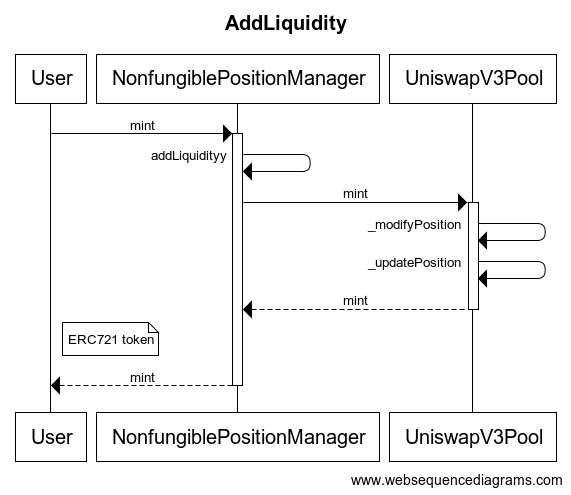struct AddLiquidityParams {
uint24 fee;         // 交易费率
int24 tickLower;    // 流动性的价格下限（以 token0 计价），这里传入的是 tick index
int24 tickUpper;    // 流动性的价格上线（以 token0 计价），这里传入的是 tick index
uint128 amount;     // 流动性 L 的值
uint256 amount0Max; // 提供的 token0 上限数
uint256 amount1Max; // 提供的 token1 上限数
}

internal
returns (
uint256 amount0,
uint256 amount1,
IUniswapV3Pool pool
)
{
PoolAddress.PoolKey({token0: params.token0, token1: params.token1, fee: params.fee});

// 这里不需要访问 factory 合约，可以通过 token0, token1, fee 三个参数计算出 pool 的合约地址

(amount0, amount1) = pool.mint(
params.recipient,
params.tickLower,
params.tickUpper,
params.amount,
// 这里是 pool 合约回调所使用的参数
abi.encode(MintCallbackData({poolKey: poolKey, payer: msg.sender}))
);

require(amount0 <= params.amount0Max);
require(amount1 <= params.amount1Max);
}


• 传入的 lower/upper 价格是以 tick index 来表示的，因此需要在链下先计算好价格所对应的 tick index

• 传入的是流动性 $L$ 的大小，这个也需要在链下先计算好，计算过程见下面

• 我们不需要访问 factory 就可以计算出 pool 的地址，实现原理见 CREATE2

• 这里有一个回调函数的参数。v3 使用回调函数来完成进行流动性 token 的支付操作，原因见下面

### 从 token 数计算流动性 L

• 当前池中的价格 $P_{c} < P_{a}$ ，如下图：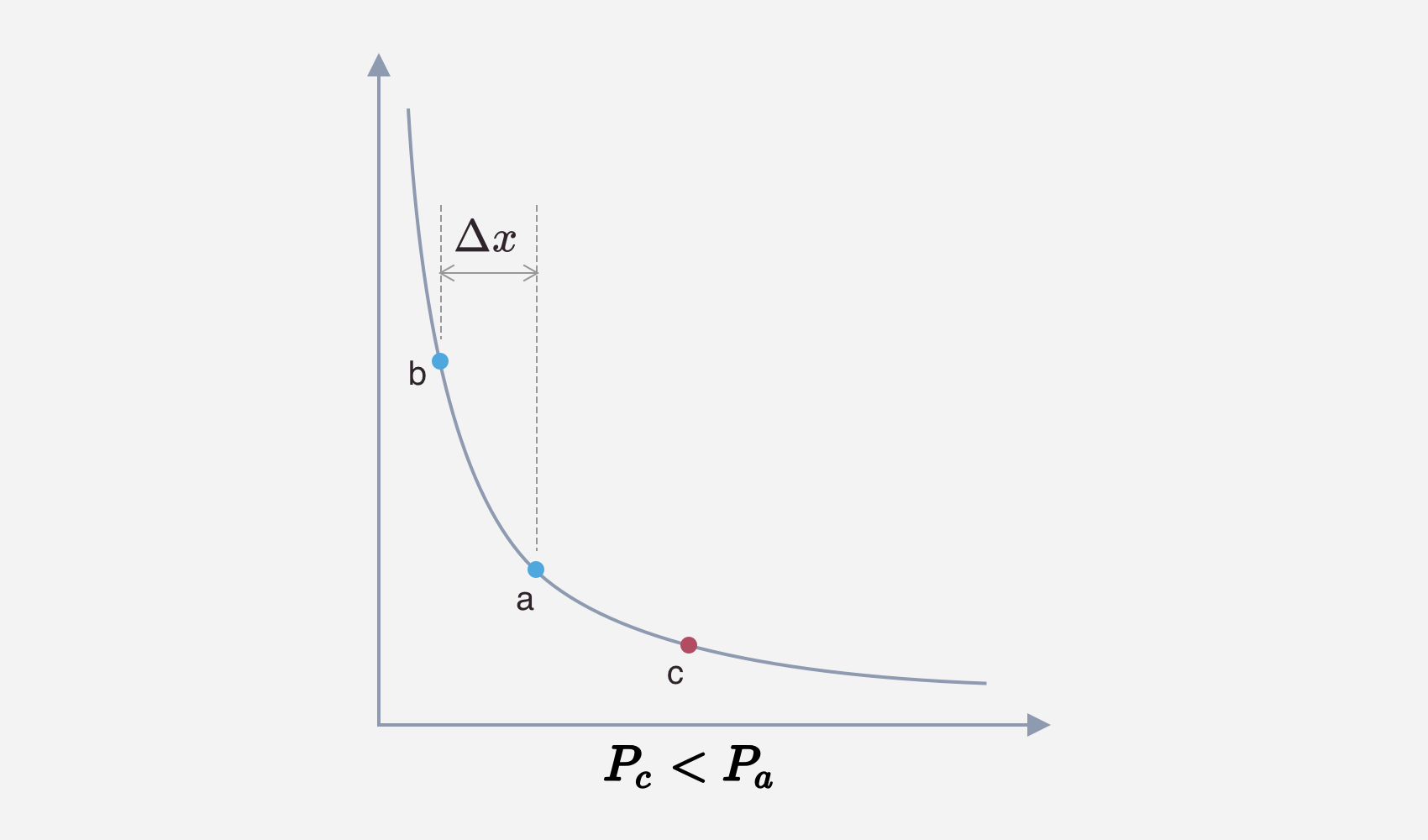$L = \frac {\Delta x}{\frac 1{\sqrt {P_{a}}} - \frac 1{\sqrt {P_{b}}}}$
• 当前池中的价格 $P_{c} > P_{b}$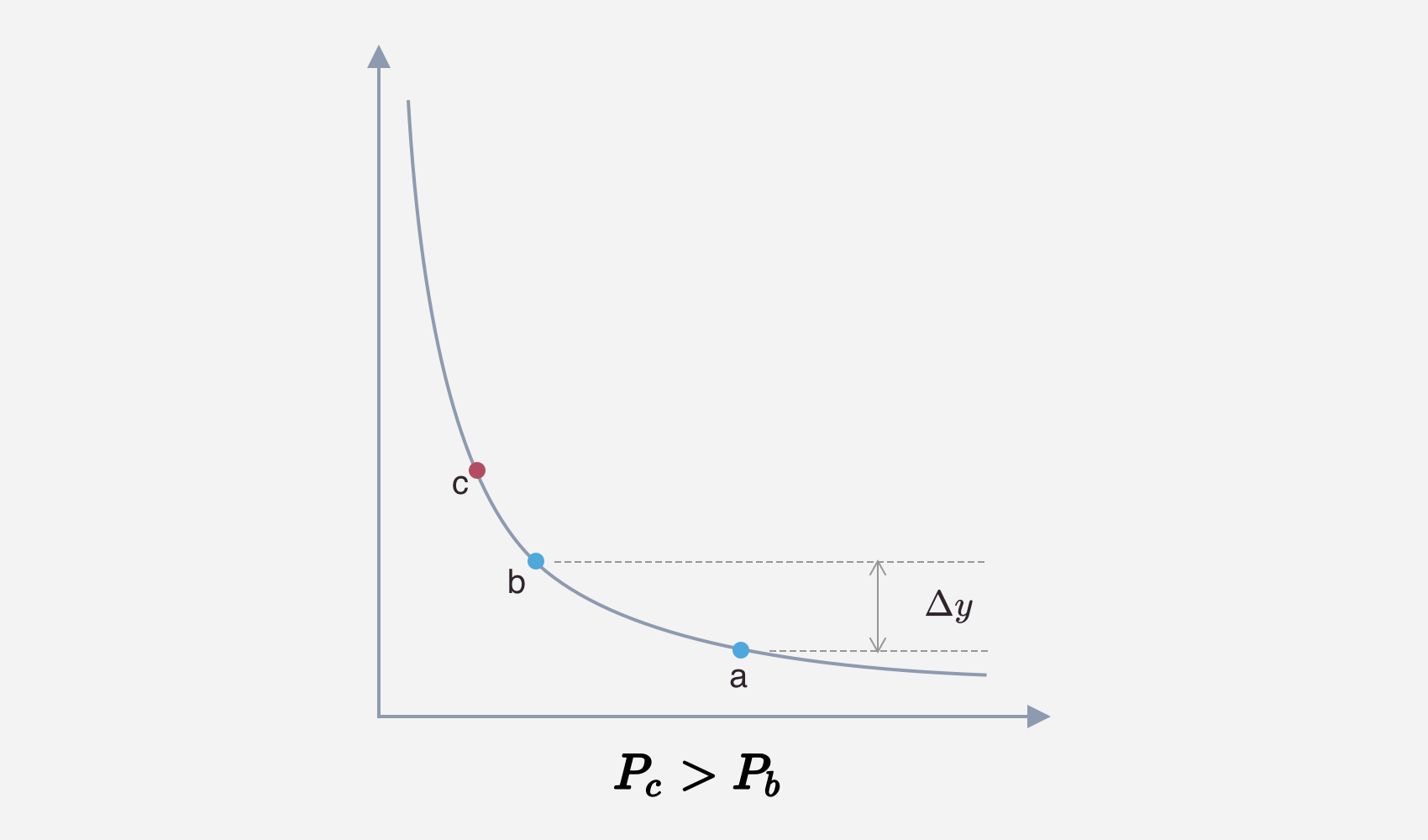$L = \frac {\Delta y}{\sqrt {P_{b}} - \sqrt {P_{a}}}$
• 当前池子中的价格 $P_{c} \in [P_{a}, P_{b}]$，如下图：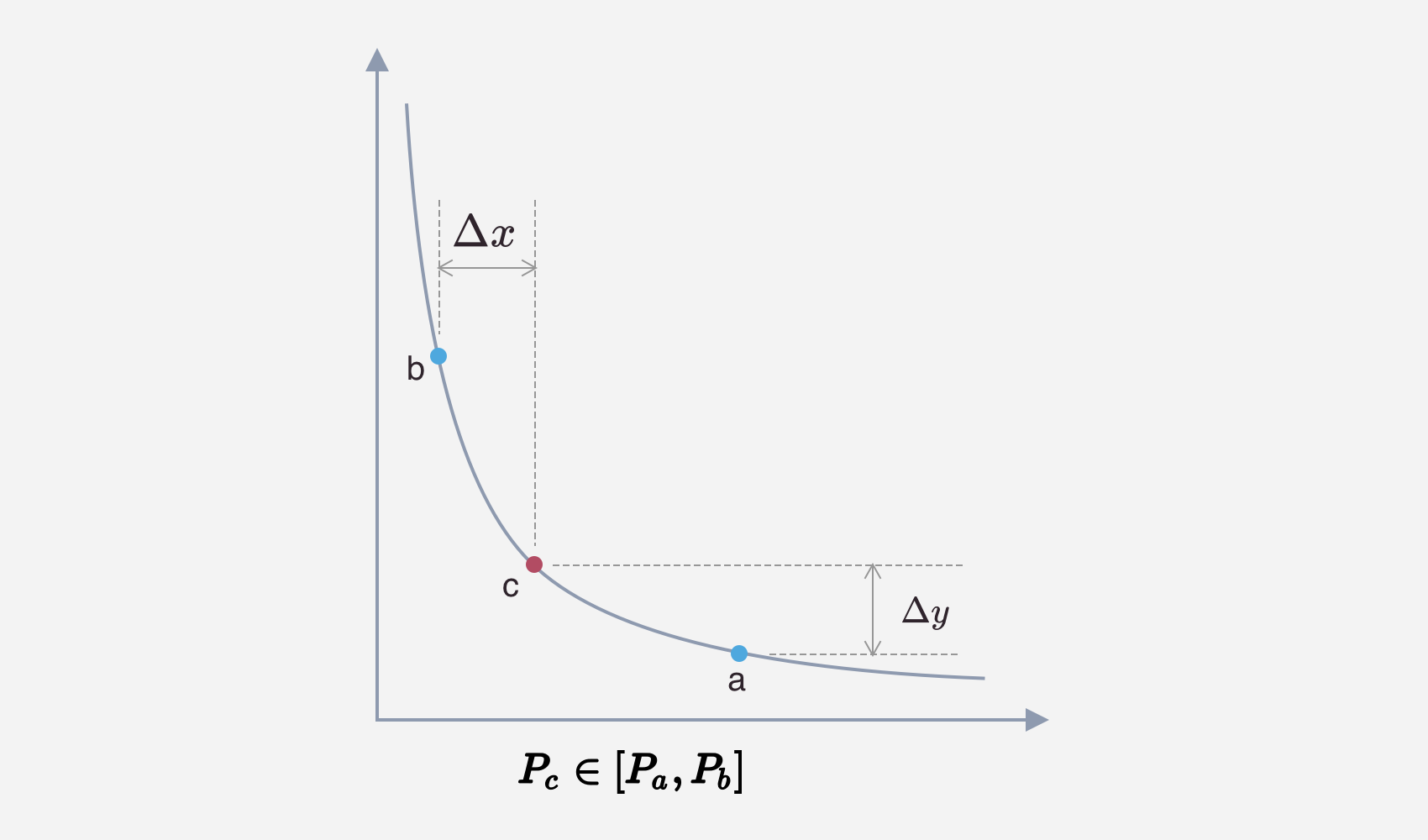$L = \frac {\Delta x}{\frac 1{\sqrt {P_{c}}} - \frac 1{\sqrt {P_{b}}}} = \frac {\Delta y}{\sqrt {P_{c}} - \sqrt {P_{a}}}$

### 回调函数

NonfungiblePositionManager 中回调函数的实现如下：

struct MintCallbackData {
address payer;         // 支付 token 的地址
}

/// @inheritdoc IUniswapV3MintCallback
function uniswapV3MintCallback(
uint256 amount0Owed,
uint256 amount1Owed,
bytes calldata data
) external override {
MintCallbackData memory decoded = abi.decode(data, (MintCallbackData));
CallbackValidation.verifyCallback(factory, decoded.poolKey);

// 根据传入的参数，使用 transferFrom 代用户向 Pool 中支付 token
if (amount0Owed > 0) pay(decoded.poolKey.token0, decoded.payer, msg.sender, amount0Owed);
if (amount1Owed > 0) pay(decoded.poolKey.token1, decoded.payer, msg.sender, amount1Owed);
}


### postion 更新

function _updatePosition(
int24 tickLower,
int24 tickUpper,
int128 liquidityDelta,
int24 tick
) private returns (Position.Info storage position) {
// 获取用户的 Postion
position = positions.get(owner, tickLower, tickUpper);
...

// 根据传入的参数修改 Position 对应的 lower/upper tick 中
// 的数据，这里可以是增加流动性，也可以是移出流动性
bool flippedLower;
bool flippedUpper;
if (liquidityDelta != 0) {
uint32 blockTimestamp = _blockTimestamp();

// 更新 lower tikc 和 upper tick
// fippedX 变量表示是此 tick 的引用状态是否发生变化，即
// 被引用 -> 未被引用 或
// 未被引用 -> 被引用
// 后续需要根据这个变量的值来更新 tick 位图
flippedLower = ticks.update(
tickLower,
tick,
liquidityDelta,
_feeGrowthGlobal0X128,
_feeGrowthGlobal1X128,
false,
maxLiquidityPerTick
);
flippedUpper = ticks.update(
tickUpper,
tick,
liquidityDelta,
_feeGrowthGlobal0X128,
_feeGrowthGlobal1X128,
true,
maxLiquidityPerTick
);

// 如果一个 tick 第一次被引用，或者移除了所有引用
// 那么更新 tick 位图
if (flippedLower) {
tickBitmap.flipTick(tickLower, tickSpacing);
secondsOutside.initialize(tickLower, tick, tickSpacing, blockTimestamp);
}
if (flippedUpper) {
tickBitmap.flipTick(tickUpper, tickSpacing);
secondsOutside.initialize(tickUpper, tick, tickSpacing, blockTimestamp);
}
}
...
// 更新 position 中的数据
position.update(liquidityDelta, feeGrowthInside0X128, feeGrowthInside1X128);

// 如果移除了对 tick 的引用，那么清除之前记录的元数据
// 这只会发生在移除流动性的操作中
if (liquidityDelta < 0) {
if (flippedLower) {
ticks.clear(tickLower);
secondsOutside.clear(tickLower, tickSpacing);
}
if (flippedUpper) {
ticks.clear(tickUpper);
secondsOutside.clear(tickUpper, tickSpacing);
}
}
}


• 添加/移除流动性时，先更新这个 Positon 对应的 lower/upper tick 中记录的元数据
• 更新 position
• 根据需要更新 tick 位图

Postion 是以 owner, lower tick, uppper tick 作为键来存储的，注意这里的 owner 实际上是 NonfungiblePositionManager 合约的地址。这样当多个用户在同一个价格区间提供流动性时，在底层的 UniswapV3Pool 合约中会将他们合并存储。而在 NonfungiblePositionManager 合约中会按用户来区别每个用户拥有的 Position.

Postion 中包含的字段中，除去费率相关的字段，只有一个即流动性 $L$：

library Position {
// info stored for each user's position
struct Info {
// 此 position 中包含的流动性大小，即 L 值
uint128 liquidity;
...
}


position.update(liquidityDelta, feeGrowthInside0X128, feeGrowthInside1X128);


### tick 管理

    // tick 元数据管理的库
using Tick for mapping(int24 => Tick.Info);
// tick 位图槽位的库
using TickBitmap for mapping(int16 => uint256);

// 记录了一个 tick 包含的元数据，这里只会包含所有 Position 的 lower/upper ticks
mapping(int24 => Tick.Info) public override ticks;
// tick 位图，因为这个位图比较长（一共有 887272x2 个位），大部分的位不需要初始化
// 因此分成两级来管理，每 256 位为一个单位，一个单位称为一个 word
// map 中的键是 word 的索引
mapping(int16 => uint256) public override tickBitmap;

library Tick {
...
// tick 中记录的数据
struct Info {
// 记录了所有引用这个 tick 的 position 流动性的和
uint128 liquidityGross;
// 当此 tick 被越过时（从左至右），池子中整体流动性需要变化的值
int128 liquidityNet;
...
}


tick 中和流动性相关的字段有两个 liquidityGrossliquidityNet

liquidityNet 表示当价格从左至右经过此 tick 时整体流动性需要变化的净值。在单个流动性中，对于 lower tick 来说，它的值为正，对于 upper tick 来说它的值为 负。

1. token0 价格上升，即从左至右越过一个 lower tick 时， $L=L_{current} + \Delta L$
2. token0 价格上升，即从左至右越过一个 upper tick 时， $L=L_{current} - \Delta L$
3. token0 价格下降，即从右至左越过一个 upper tick 时， $L=L_{current} + \Delta L$
4. token0 价格下降，即从右至左越过一个 lower tick 时， $L=L_{current} - \Delta L$

liquidityNet 中记录的就是当从左至右穿过这个 tick 时，需要增减的流动性，当其为 lower tick 时，其值为正，当其为 upper tick 时，其值为负。对于从右至左穿过的情况，只需将 liquidityNet 的值去翻即可完成计算。

function update(
mapping(int24 => Tick.Info) storage self,
int24 tick,
int24 tickCurrent,
int128 liquidityDelta,
uint256 feeGrowthGlobal0X128,
uint256 feeGrowthGlobal1X128,
bool upper,
uint128 maxLiquidity
) internal returns (bool flipped) {
Tick.Info storage info = self[tick];

uint128 liquidityGrossBefore = info.liquidityGross;

require(liquidityGrossAfter <= maxLiquidity, 'LO');

// 通过 liquidityGross 在进行 position 变化前后的值
// 来判断 tick 是否仍被引用
flipped = (liquidityGrossAfter == 0) != (liquidityGrossBefore == 0);

...

info.liquidityGross = liquidityGrossAfter;

// 更新 liquidityNet 的值，对于 upper tick，
info.liquidityNet = upper
? int256(info.liquidityNet).sub(liquidityDelta).toInt128()
}


### tick 位图

tick 位图用于记录所有被引用的 lower/upper tick index，我们可以用过 tick 位图，从当前价格找到下一个（从左至右或者从右至左）被引用的 tick index。关于 tick 位图的管理，在 _updatePosition 中的：

if (flippedLower) {
tickBitmap.flipTick(tickLower, tickSpacing);
secondsOutside.initialize(tickLower, tick, tickSpacing, blockTimestamp);
}
if (flippedUpper) {
tickBitmap.flipTick(tickUpper, tickSpacing);
secondsOutside.initialize(tickUpper, tick, tickSpacing, blockTimestamp);
}


• 对于不存在的 tick，不需要初始值，因为访问 map 中不存在的 key 默认值就是 0
• 通过对位图的每个 word(uint256) 建立索引来管理位图，即访问路径为 word index -> word -> tick bit

### token 数确认

_modifyPosition 函数在调用 _updatePosition 更新完 Position 后，会计算出此次提供流动性具体所需的 x token 和 y token 数量。

function _modifyPosition(ModifyPositionParams memory params)
private
noDelegateCall
returns (
Position.Info storage position,
int256 amount0,
int256 amount1
)
{
...
Slot0 memory _slot0 = slot0; // SLOAD for gas optimization

position = _updatePosition(
...
);

...
}


Slot0 memory _slot0 = slot0; // SLOAD for gas optimization


function _modifyPosition(ModifyPositionParams memory params)
private
noDelegateCall
returns (
Position.Info storage position,
int256 amount0,
int256 amount1
)
{
...

if (params.liquidityDelta != 0) {
// 计算三种情况下 amount0 和 amount1 的值，即 x token 和 y token 的数量
if (_slot0.tick < params.tickLower) {
amount0 = SqrtPriceMath.getAmount0Delta(
// 计算 lower/upper tick 对应的价格
TickMath.getSqrtRatioAtTick(params.tickLower),
TickMath.getSqrtRatioAtTick(params.tickUpper),
params.liquidityDelta
);
} else if (_slot0.tick < params.tickUpper) {
// current tick is inside the passed range
uint128 liquidityBefore = liquidity; // SLOAD for gas optimization

...

amount0 = SqrtPriceMath.getAmount0Delta(
_slot0.sqrtPriceX96,
TickMath.getSqrtRatioAtTick(params.tickUpper),
params.liquidityDelta
);
amount1 = SqrtPriceMath.getAmount1Delta(
TickMath.getSqrtRatioAtTick(params.tickLower),
_slot0.sqrtPriceX96,
params.liquidityDelta
);

} else {
amount1 = SqrtPriceMath.getAmount1Delta(
TickMath.getSqrtRatioAtTick(params.tickLower),
TickMath.getSqrtRatioAtTick(params.tickUpper),
params.liquidityDelta
);
}
}
}


1. 当提供/增加流动性时，会使用 RoundUp，这样可以保证增加数量为 L 的流动性时，用户提供足够的 token 到 pool 中
2. 当移除/减少流动性时，会使用 RoundDown，这样可以保证减少数量为 L 的流动性时，不会从 pool 中给用户多余的 token

### tick index -> $\sqrt P$

function getSqrtRatioAtTick(int24 tick) internal pure returns (uint160 sqrtPriceX96) {
uint256 absTick = tick < 0 ? uint256(-int256(tick)) : uint256(int256(tick));
require(absTick <= uint256(MAX_TICK), 'T');

// 这些魔数分别表示 1/sqrt(1.0001)^1, 1/sqrt(1.0001)^2, 1/sqrt(1.0001)^4....
uint256 ratio = absTick & 0x1 != 0 ? 0xfffcb933bd6fad37aa2d162d1a594001 : 0x100000000000000000000000000000000;
if (absTick & 0x2 != 0) ratio = (ratio * 0xfff97272373d413259a46990580e213a) >> 128;
if (absTick & 0x4 != 0) ratio = (ratio * 0xfff2e50f5f656932ef12357cf3c7fdcc) >> 128;
if (absTick & 0x8 != 0) ratio = (ratio * 0xffe5caca7e10e4e61c3624eaa0941cd0) >> 128;
if (absTick & 0x10 != 0) ratio = (ratio * 0xffcb9843d60f6159c9db58835c926644) >> 128;
if (absTick & 0x20 != 0) ratio = (ratio * 0xff973b41fa98c081472e6896dfb254c0) >> 128;
if (absTick & 0x40 != 0) ratio = (ratio * 0xff2ea16466c96a3843ec78b326b52861) >> 128;
if (absTick & 0x80 != 0) ratio = (ratio * 0xfe5dee046a99a2a811c461f1969c3053) >> 128;
if (absTick & 0x100 != 0) ratio = (ratio * 0xfcbe86c7900a88aedcffc83b479aa3a4) >> 128;
if (absTick & 0x200 != 0) ratio = (ratio * 0xf987a7253ac413176f2b074cf7815e54) >> 128;
if (absTick & 0x400 != 0) ratio = (ratio * 0xf3392b0822b70005940c7a398e4b70f3) >> 128;
if (absTick & 0x800 != 0) ratio = (ratio * 0xe7159475a2c29b7443b29c7fa6e889d9) >> 128;
if (absTick & 0x1000 != 0) ratio = (ratio * 0xd097f3bdfd2022b8845ad8f792aa5825) >> 128;
if (absTick & 0x2000 != 0) ratio = (ratio * 0xa9f746462d870fdf8a65dc1f90e061e5) >> 128;
if (absTick & 0x4000 != 0) ratio = (ratio * 0x70d869a156d2a1b890bb3df62baf32f7) >> 128;
if (absTick & 0x8000 != 0) ratio = (ratio * 0x31be135f97d08fd981231505542fcfa6) >> 128;
if (absTick & 0x10000 != 0) ratio = (ratio * 0x9aa508b5b7a84e1c677de54f3e99bc9) >> 128;
if (absTick & 0x20000 != 0) ratio = (ratio * 0x5d6af8dedb81196699c329225ee604) >> 128;
if (absTick & 0x40000 != 0) ratio = (ratio * 0x2216e584f5fa1ea926041bedfe98) >> 128;
if (absTick & 0x80000 != 0) ratio = (ratio * 0x48a170391f7dc42444e8fa2) >> 128;

if (tick > 0) ratio = type(uint256).max / ratio;

// this divides by 1<<32 rounding up to go from a Q128.128 to a Q128.96.
// we then downcast because we know the result always fits within 160 bits due to our tick input constraint
// we round up in the division so getTickAtSqrtRatio of the output price is always consistent
sqrtPriceX96 = uint160((ratio >> 32) + (ratio % (1 << 32) == 0 ? 0 : 1));
}


$\sqrt P_i = {\sqrt {1.0001}}^i$

$\begin{cases} i = n_0 \cdot 1 + n_1 \cdot 2 + n_2 \cdot 4 + n_3 \cdot 8 + .... \\ n_i \in \{0, 1\} \end{cases}$

$\begin{cases} \sqrt P = {\sqrt {1.0001}}^i = {\sqrt {1.0001}}^{n_0 \cdot 1} \cdot {\sqrt {1.0001}}^{n_1 \cdot 2} \cdot {\sqrt {1.0001}}^{n_2 \cdot 4} \cdot {\sqrt {1.0001}}^{n_3 \cdot 8} \cdot ... \\ n_i \in \{0, 1\} \end{cases}$

• 因为 $\sqrt {P_{-i}} = \frac 1{\sqrt P_{i}}$，所以当 $i$ 为负数时可以先将其取反，转换为正数进行计算
• 当 $i$ 的值为正数时，计算的结果可能会很大，中间计算涉及到很多乘法运算，可能会导致计算结果溢出（它使用了 Q128.128 定点数）。所以实际计算的是 $i$ 为负数时的值，因为当 $i$ 为负数时，$\sqrt P_{i}$ 是一个小于 1 的小数，这样在进行乘法运算时则不会产生溢出。即上面代码的那些魔数分别为 $\frac 1{\sqrt {1.0001}^1},\ \frac 1{\sqrt {1.0001}^2},\ \frac 1{\sqrt {1.0001}^4},\ \frac 1{\sqrt {1.0001}^8},\ …,\ \frac 1{\sqrt {1.0001}^{524288}}$ 的值
• 这里的计算使用了 Q128.128 精度的定点数，实际上这些魔数的值都向右移动 128 位
• 最后，当输入是正数时，我们需要在计算的结尾计算 $\sqrt P_{i} = \frac 1{\sqrt P_{-i}}$，即 $\sqrt P_{i}$ 的倒数，这里因为使用了 Q128.128 精度的定点数，即计算的过程是： $\frac {1«128}{\sqrt P_{-i}} « 128 = \frac {1«256}{\sqrt P_{-i}}$，1<<256 可以使用 type(uint256).max 取近似值来表示
• 最后的最后，将 Q128.128 转换为 Q64.96 并始终向上取整，以保持一致性

### 完成流动性添加

_modifyPosition 调用完成后，会返回 x token, 和 y token 的数量。再来看 UniswapV3Pool.mint 的代码：

function mint(
int24 tickLower,
int24 tickUpper,
uint128 amount,
bytes calldata data
) external override lock returns (uint256 amount0, uint256 amount1) {
require(amount > 0);
(, int256 amount0Int, int256 amount1Int) =
_modifyPosition(
ModifyPositionParams({
owner: recipient,
tickLower: tickLower,
tickUpper: tickUpper,
liquidityDelta: int256(amount).toInt128()
})
);

amount0 = uint256(amount0Int);
amount1 = uint256(amount1Int);

uint256 balance0Before;
uint256 balance1Before;
// 获取当前池中的 x token, y token 余额
if (amount0 > 0) balance0Before = balance0();
if (amount1 > 0) balance1Before = balance1();
// 将需要的 x token 和 y token 数量传给回调函数，这里预期回调函数会将指定数量的 token 发送到合约中
IUniswapV3MintCallback(msg.sender).uniswapV3MintCallback(amount0, amount1, data);
// 回调完成后，检查发送至合约的 token 是否复合预期，如果不满足检查则回滚交易
if (amount0 > 0) require(balance0Before.add(amount0) <= balance0(), 'M0');
if (amount1 > 0) require(balance1Before.add(amount1) <= balance1(), 'M1');

emit Mint(msg.sender, recipient, tickLower, tickUpper, amount, amount0, amount1);
}


function mint(MintParams calldata params)
external
payable
override
returns (
uint256 tokenId,
uint256 amount0,
uint256 amount1
)
{
IUniswapV3Pool pool;
// 这里是添加流动性，并完成 x token 和 y token 的发送
token0: params.token0,
token1: params.token1,
fee: params.fee,
tickLower: params.tickLower,
tickUpper: params.tickUpper,
amount: params.amount,
amount0Max: params.amount0Max,
amount1Max: params.amount1Max
})
);

// 铸造 ERC721 token 给用户，用来代表用户所持有的流动性
_mint(params.recipient, (tokenId = _nextId++));

bytes32 positionKey = PositionKey.compute(address(this), params.tickLower, params.tickUpper);
(, uint256 feeGrowthInside0LastX128, uint256 feeGrowthInside1LastX128, , ) = pool.positions(positionKey);

// idempotent set
uint80 poolId =
cachePoolKey(
PoolAddress.PoolKey({token0: params.token0, token1: params.token1, fee: params.fee})
);

// 用 ERC721 的 token ID 作为键，将用户提供流动性的元信息保存起来
_positions[tokenId] = Position({
nonce: 0,
poolId: poolId,
tickLower: params.tickLower,
tickUpper: params.tickUpper,
liquidity: params.amount,
feeGrowthInside0LastX128: feeGrowthInside0LastX128,
feeGrowthInside1LastX128: feeGrowthInside1LastX128,
tokensOwed0: 0,
tokensOwed1: 0
});
}


### 流动性的移除

function burn(
int24 tickLower,
int24 tickUpper,
uint128 amount
) external override lock returns (uint256 amount0, uint256 amount1) {
// 先计算出需要移除的 token 数
(Position.Info storage position, int256 amount0Int, int256 amount1Int) =
_modifyPosition(
ModifyPositionParams({
owner: msg.sender,
tickLower: tickLower,
tickUpper: tickUpper,
liquidityDelta: -int256(amount).toInt128()
})
);

amount0 = uint256(-amount0Int);
amount1 = uint256(-amount1Int);

// 注意这里，移除流动性后，将移出的 token 数记录到了 position.tokensOwed 上
if (amount0 > 0 || amount1 > 0) {
(position.tokensOwed0, position.tokensOwed1) = (
position.tokensOwed0 + uint128(amount0),
position.tokensOwed1 + uint128(amount1)
);
}

emit Burn(msg.sender, tickLower, tickUpper, amount, amount0, amount1);
}


## Uniswap v3 详解系列